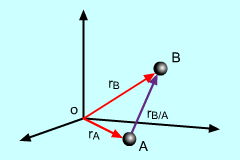Ch 1. Particle General Motion Multimedia Engineering Dynamics Position,Vel & Accel. Accel. varyw/ Time Accel. Constant Rect. Coordinates Norm/Tang. Coordinates Polar Coordinates RelativeMotion
 Chapter - Particle - 1. General Motion 2. Force & Accel. 3. Energy 4. Momentum - Rigid Body - 5. General Motion 6. Force & Accel. 7. Energy 8. Momentum 9. 3-D Motion 10. Vibrations Appendix Basic Math Units Basic Equations Sections Search eBooks Dynamics Fluids Math Mechanics Statics Thermodynamics Author(s): Kurt Gramoll ©Kurt GramollDYNAMICS - THEORYIn many situations, there is a need to examine the motion of two or more points relative to each other.

Consider two points, A and B, both in motion relative to the origin O. This section will examine the motion of B relative to A.

Position

If rB/A is the position vector of B relative to A, then it can be stated that
 rB = rA + rB/A

This is simply adding two position vectors to get a new total vector. This is illustrated in the diagram at the left.

Velocity

To find the relative velocities of two points, take the time derivative of the position, giving

d(rB)/dt = d(rA + rB/A)/dt

 vB = vA + vB/A

Acceleration

Relative acceleration is found by taking the time derivative of the velocity,

d(vB)/dt = d(vA + vB/A)/dt

 aB = aA + aB/A

Practice Homework and Test problems now available in the 'Eng Dynamics' mobile app
Includes over 400 problems with complete detailed solutions.
Available now at the Google Play Store and Apple App Store.